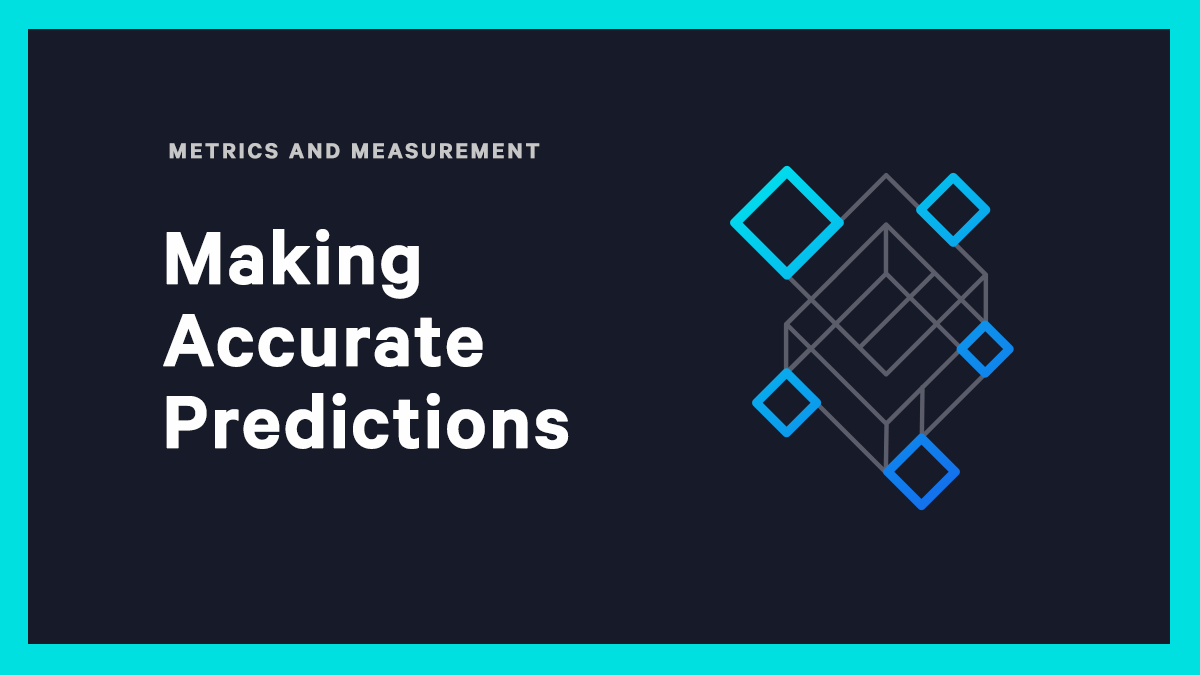xBroken Lifecycle

# Making Accurate Predictions

by
Andrew Engel
on
5/7/2022In last week's blog, I discussed a problem with Churn modeling that I regularly ran across when discussing marketing analytics problems with various data science teams. This week I’d like to discuss another common issue I ran across involving metrics used to evaluate binary classification machine learning models. Too often, data science teams, when discussing their models, would refer to those statistics that depend on statistics derived by predicting which class an observation belongs to, such as accuracy, precision or sensitivity.

Why is this a problem?  There are several reasons. Machine learning algorithms are not predicting the class directly, rather they are predicting a number between zero and one that we can map to be the probability of being in class one. This means that in order to predict the class, we need to define a threshold between zero and one and define the class prediction as

However, more than half the time, when I ask what threshold they chose, the data science team doesn’t even understand the question. They simply called predict() on the model they built and don’t realize that this method assumes a threshold of 0.5. This threshold may not even give the best accuracy for their given model. As an example, we can create a test dataset with scikit-learn as follows.

X, y = skd.make_classification(n_samples=250000,
n_features=100,
n_informative=50,
n_redundant=25,
n_classes=2,
n_clusters_per_class=20,
flip_y=.2,
weights=[.5, .5],
hypercube=False,
random_state=1666)

We can then build a model with catboos, and use predict_proba() to get the probabilities of each record in a validation set and step through each possible threshold in increments of 0.01.

tmp_pred = model.predict_proba(X_test)

prob_pred = tmp_pred[:,1]
for t in range(0, 100):
threshold = t/100.0
pred = (prob_pred >= threshold).astype('int')
accuracy = skm.accuracy_score(y_test, pred)
f1 = skm.f1_score(y_test, pred)
precision = skm.precision_score(y_test, pred)
recall = skm.recall_score(y_test, pred)

The model accuracy using predict() was 0.70176 and this was also the accuracy with a threshold of 0.5 in the code above. The maximum accuracy was 0.70186 with a threshold of 0.51. Not a huge difference admittedly, but enough to suggest that data scientists shouldn’t take the results of predict() without considering other thresholds.

If we create an unbalanced data set with 33% in one class and 67% in the other class, the difference becomes larger. The accuracy of predict() and a threshold of 0.50 is 0.71662, while the maximum accuracy occurs at a threshold of 0.54 giving an accuracy of 0.72148, nearly a percent better performance.

This doesn’t even begin to get into the major problems with accuracy as a metric. First, rarely are the classes equally important to the business decision. In the churn case, it is often less costly to incorrectly classify non-churning customers as churn and take action to retain that customer than it is to miss churning customers and have them churn. In this case, other metrics such as false negative rate may matter most of all. In other cases, precision or sensitivity may be the best judge of model performance. However, it is unlikely that any of these metrics will be maximized with a threshold of 0.5.

Beyond all of this, accuracy is a horrible metric to evaluate a model on. Let’s consider a simple example of two models and their predictions.

Using a threshold of 0.5, we see that the models get the same observations correct and incorrect.

These two models have the same accuracy, and in fact the same recall, precision, etc., but do we really think these models are equivalent? I strongly prefer model 1 and would put it into production over model 2. When model 1 is right, it is confident with predictions close to one when true and zero when false. Further, when it is wrong, it is unsure with predictions close to 0.5. On the other hand, model 2 is confident when it is wrong and unsure when it is right. If we use a metric like log loss that captures this certainty along with the correctness we can easily identify that model 1 is the better model.

Too often in my career, I have spoken to data scientists who are evaluating models based on accuracy or other similar metrics, but haven’t worried about the threshold they were using. When evaluating models, it is necessary to consider the problem you are trying to solve, the business needs and to use metrics that properly measure the performance of the model.